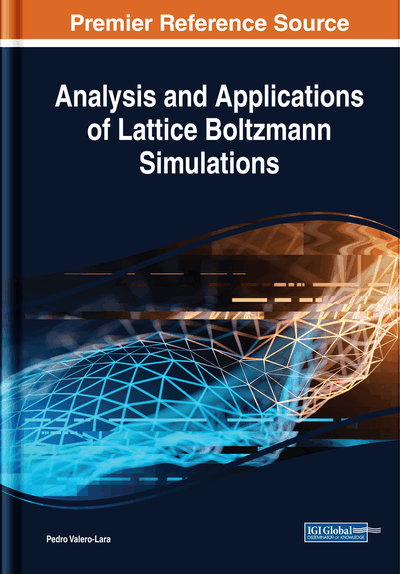# Fluid-Structure Interaction Using Lattice Boltzmann Method Coupled With Finite Element Method

Zhe Li (Ecole Centrale de Nantes, France) and Julien Favier (Aix-Marseille University, France)
DOI: 10.4018/978-1-5225-4760-0.ch008
Available
\$37.50
No Current Special Offers

## Abstract

This chapter presents several partitioned algorithms to couple lattice Boltzmann method (LBM) and finite element method (FEM) for numerical simulation of transient fluid-structure interaction (FSI) problems with large interface motion. Partitioned coupling strategies allow one to solve separately the fluid and solid subdomains using adapted or optimized numerical schemes, which provides a considerable flexibility for FSI simulation, especially for more realistic and industrial applications. However, partitioned coupling procedures often encounter numerical instabilities due to the fact that the time integrations of the two subdomains are usually carried out in a staggered way. As a consequence, the energy transfer across the fluid-solid interface is usually not correctly simulated, which means numerical energy injection or dissipation might occur at the interface with partitioned methods. The focus of the present chapter is given to the energy conservation property of different partitioned coupling strategies for FSI simulation.
Chapter Preview
Top

## Introduction

Fluid-Structure Interaction (FSI) can be usually observed in nature and engineering processes. Well-known examples include wind-induced oscillations of tall buildings, collapse of bridges caused by wind gusts, flow-induced vibrations of airfoils and dynamics of blood flow through valves in veins etc. The FSI effects are interesting and of great importance, because in some cases they are the key points to understand well the underlying physical phenomenon.

Although experimental results may offer the most straightforward and effective conclusions on some FSI problems, the experimentations are often limited by some constraints, such as the choice of dominant dimensionless number, the high cost of the materials and the long period of test etc. With the development of High Performance Computation (HPC), numerical simulation becomes a useful tool which is more and more efficient and powerful for analyzing the FSI problems. Thus, the robustness and accuracy of the numerical methods for their simulations are of primary importance.

Due to the different material’s characteristics of fluid and solid media, FSI simulations are often carried out with different numerical methods for the two individual sub-domains, i.e. fluid and solid. In such circumstance, a partitioned coupling procedure is often preferred, comparing with a monolithic coupling procedure. Because partitioned coupling procedures allow one to carry out separately the time integration of each sub-domain, unlike the monolithic ones which treat computationally the fluid and solid sub-domains as an entity (Felippa, Park, & Farhat, 2001). This is particularly convenient when one prefers to solve the fluid and solid equations using different numerical methods or existing softwares, which are already designed and optimized for each individual sub-domain (Farhat, van der Zee, & Geuzaine, 2006).

However, partitioned coupling procedures often suffer from numerical instabilities, if no special synchronization techniques, such as sub-iterative methods (Farhat, Rallu, Wang, & Belytschko, 2010) or structural predictors (Piperno, & Farhat, 2001), are applied. This is because in this kind of partitioned coupling procedure, the time integrations of the fluid and solid sub-domains are carried out in a staggered way, which means that one updates separately the fluid and solid status to the next time-instant, by introducing some approximations. For example, when updating the solid status, a usual approximation is to assume that the flow-induced pressure loading is constant during one time-step and it is equal to the pressure at the previous time-instant. With this kind of partitioned coupling scheme, named as Ordinary Staggered Coupling Scheme (OSCS) hereafter, the FSI computations may diverge rapidly, even though the individual schemes used in the fluid and solid sub-domains are both numerically stable. This is mainly due to the time-lag between the time integrations of the fluid and solid sub-domains (Michler, Hulshoff, van Brummelen, & de Borst, 2004). From the energy point of view, this OSCS algorithmically injects or dissipates energy at the fluid-solid interface. When too much algorithmic interface energy is injected to the system, the coupling simulation will be interrupted due to the numerical instability. Hence this chapter will give the focus on the interface-energy-conserving property of different partitioned coupling algorithms for FSI simulations by coupling Lattice Boltzmann Method (LBM) for fluid and Finite Element Method (FEM) for solid.

The fluid sub-domain is simulated with the LBM, which is gaining more and more attention over the past two decades. As an alternative to conventional numerical solvers based on the resolution of Navier-Stokes (NS) equations, LBM consists in solving the discrete Boltzmann equation with collision and streaming processes, which can be proven to fully recover the macroscopic NS equations through the Chapman-Enskog analysis (Chapman, & Cowling, 1970). Recently, Shan et al. (Shan, Yuan, & Chen, 2006) have shown that the LBM can be systematically derived from the kinetic theory of hydrodynamics. In this chapter, a single-component isothermal LBM model is applied for the simulation of a weakly compressible laminar fluid flow interacted with a deformable solid structure which is simulated by means of FEM.

## Complete Chapter List

Search this Book:
Reset